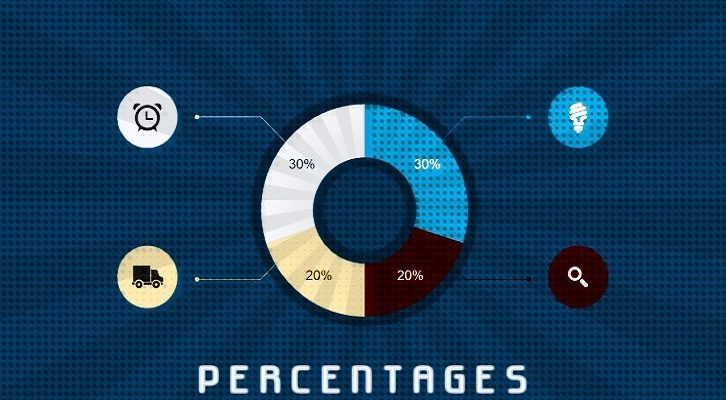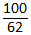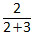# CAT – Percentages

### Percentages Question

Question:
An alloy of copper and aluminum has 40% copper. An alloy of Copper and Zinc has Copper and Zinc in the ratio 2: 7. These two alloys are mixed in such a way that in the overall alloy, there is more aluminum than Zinc, and copper constitutes x% of this alloy. What is the range of values x can take?
A.  30% < x < 40%
B.  32.5% < x < 42%
C.  33.33% < x < 40%
D.  32.5% < x < 40%
Correct Answer : (A)
Explanation:
 Alloy 1 Copper Aluminium 2x 3x Alloy 2 Copper Zinc 2y 7y
If Aluminium and Zinc have to be equal, 3x = 7y. The simplest case occurs at the LCM, when both Aluminium and Zinc are 21kg.
 Alloy 1 Copper Aluminium 14 21 Alloy 2 Copper Zinc 6 21
Even a gram of alloy 1 above this level would mean that there is more Aluminium than Zinc in the total alloy. So, this table is a limit on the percentages of different metals.
The percentage of Copper in the total alloy would be (14 + 6)*= 32.25%
On the other hand, if we want more Aluminium than Zinc, we can use tons and tons of alloy 1, and only a microgram of Alloy 2. The quantity of alloy 2 can be made so small that its presence can be almost neglected, as it will have as good as zero impact on the overall percentage of copper. This is another extreme case, in which Copper will have 40% weight in the alloy.
Thus, the percentage of Copper ranges from 32.25% to 40% in this alloy.
Answer Choice (A)
Level of difficulty 2